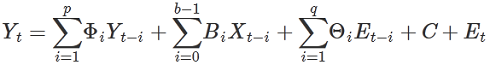# 7.00.02 - VARMAX - Aster Analytics

## Teradata Aster® Analytics Foundation User GuideUpdate 2

prodname
Aster Analytics
vrm_release
7.00.02
created_date
September 2017
category
Programming Reference
User Guide
featnum
B700-1022-700K

VARMAX (Vector Autoregressive Moving Average model with eXogenous variables) extends the ARMA/ARIMA model in two ways:

• To work with time series with multiple response variables (vector time series).
• To work with exogenous variables, or variables that are independent of the other variables in the system.

The model includes both the dynamic relationship between the multiple response variables and the relationship between the dependent and independent variables.

This formula represents a nonseasonal VARMAX model:In the preceding equation, Y t is a stationarized time series. The first term is the autoregressive component, the second term is the exogenous component, the third term is the moving average component, the fourth (C) is a vector of constants, and the fifth (E t ) is a vector of residual errors, and:

• Y t is a vector of n response variables
• X t is a vector of m exogenous variables
• p is the number of previous periods of the endogenous variables included in the model
• q is the number of previous periods included in the moving average
• b is the number of previous periods of exogenous variables included
• Φ i is an n * n matrix of autoregressive parameters
• B i is an n * m matrix of exogenous variable parameters
• Θ i is an n * n matrix of moving average parameters
• E t is the difference between the actual and the predicted value of Y t , (Y t - Ŷ t ).

This formula represents a seasonal VARMAX model:

(1 - Φ1Back - … - Φ p Back p )(1 - Φ1Back m - … - Φ sp Back m*sp )(1 - Back) d (1 - Back m ) sd (Y t ) =

C + (1 + Θ1Back + … + Θ q Back q ) (1 + Θ1Back m + … + Θ sq Back m*sq ) E t +

(B 1 + B 2Back + … + B b-1Back b ) (1 - Back) d (1 - Back m ) sd X t

Where the variables are as previously described and:

• Back is the backshift operator, that is:

Back(y t ) = y t-1

Back n (y t ) = y t-n

• m is the number of periods per season
• d is the number of differencing steps performed to stationarize the time series
• sp, sq, sd are the seasonal parameters corresponding to p, q, d
The results of the VARMAX function and the VARMA function in the R package MTS might not match exactly, because VARMA applies a boundary to the numerical optimization and VARMAX does not. Also, the default optimization method (the Method argument) is different for VARMA and VARMAX.